# Block diagram showing how to turn white noise into rain

Summary:
block diagram how to turn white noise in to rain
So I have been looking and trying different thing to synthesize rain noise like this: https://mynoise.net/NoiseMachines/campingRainNoiseGenerator.php

but I cannot get it.

and the graph that this sound makes did not help at all: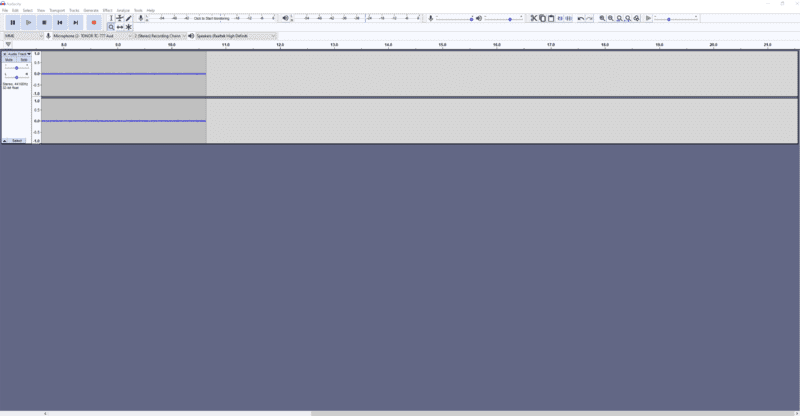can some make a block diagram of how to do this?

Baluncore
The thing about rain drops is that they are all of similar size, falling at similar speeds.
The pulses are more like shot noise than white noise.
The fundamental random feature is the time of arrival.

To generate the drum sound of a raindrop impact on a tent fabric you need to trigger the drum at random times. When a raindrop hits a taught fabric, it plucks a narrow band tuned circuit that rings with a Q of about 5 or 10 = cycles. The centre frequency of the plucked filter is dependent on tension in fabric.

If you start with white noise you must convert that to random trigger events. Maybe use a comparator that detects when the random noise amplitude is greater than a set value. The positive edge of that triggers the drum filter.

You do not identify your simulation software.

••hutchphd and Klystron
berkeman
Mentor
What do you mean by "diagram"? What kind of diagram? Like a diagram in Labview or something?

Rain noise is a mix of several types of randomness, so it will likely take a few lines of code to synthesize it. You've mentioned 44100Hz in your other thread -- do you have experience generating *.wav files?

https://en.wikipedia.org/wiki/44,100_Hz

EDIT -- Darn it, @Baluncore beat me to the punch again!

Last edited:
What do you mean by "diagram"? What kind of diagram? Like a diagram in Labview or something?

Rain noise is a mix of several types of randomness, so it will likely take a few lines of code to synthesize it. You've mentioned 44100Hz in your other thread -- do you have experience generating *.wav files?

https://en.wikipedia.org/wiki/44,100_Hz

EDIT -- Darn it, @Baluncore beat me to the punch again!
Sorry, I meant block diagram. I do not know Labview ... lol

Yes I do .
check this out :
https://www.amazon.com/dp/B01MS8W9XI/?tag=pfamazon01-20

berkeman
Mentor
To generate the drum sound of a raindrop impact on a tent fabric you need to trigger the drum at random times. When a raindrop hits a taught fabric, it plucks a narrow band tuned circuit that rings with a Q of about 5 or 10 = cycles. The centre frequency of the plucked filter is dependent on tension in fabric.

If you start with white noise you must convert that to random trigger events. Maybe use a comparator that detects when the random noise amplitude is greater than a set value. The positive edge of that triggers the drum filter.

You do not identify your simulation software.

The thing about rain drops is that they are all of similar size, falling at similar speeds.
The pulses are more like shot noise than white noise.
The fundamental random feature is the time of arrival.

To generate the drum sound of a raindrop impact on a tent fabric you need to trigger the drum at random times. When a raindrop hits a taught fabric, it plucks a narrow band tuned circuit that rings with a Q of about 5 or 10 = cycles. The centre frequency of the plucked filter is dependent on tension in fabric.

If you start with white noise you must convert that to random trigger events. Maybe use a comparator that detects when the random noise amplitude is greater than a set value. The positive edge of that triggers the drum filter.

You do not identify your simulation software.
Sorry , I am just seeing this, the one is the picture above is audacity.
https://www.audacityteam.org/

The thing about rain drops is that they are all of similar size, falling at similar speeds.
The pulses are more like shot noise than white noise.
The fundamental random feature is the time of arrival.

To generate the drum sound of a raindrop impact on a tent fabric you need to trigger the drum at random times. When a raindrop hits a taught fabric, it plucks a narrow band tuned circuit that rings with a Q of about 5 or 10 = cycles. The centre frequency of the plucked filter is dependent on tension in fabric.

If you start with white noise you must convert that to random trigger events. Maybe use a comparator that detects when the random noise amplitude is greater than a set value. The positive edge of that triggers the drum filter.

You do not identify your simulation software.
Wait are you saying that there should be time where the generate graph is 0 then randomly the sound should spike up . what is the drum filter ?

dlgoff
Gold Member
... I have been looking and trying different thing to synthesize rain noise ...

I was fortunate enough to attend an Electrical Engineering lecture by Robert Moog back in the 1960's. He used all analog circuitry. I was inspired by him and made my own synthesizer that simulated stringed instruments. If I remember correctly, it had some random noise generators that worked well for making snare drum audio, etc. Have you considered doing it with analog circuitry?

I was fortunate enough to attend an Electrical Engineering lecture by Robert Moog back in the 1960's. He used all analog circuitry. I was inspired by him and made my own synthesizer that simulated stringed instruments. If I remember correctly, it had some random noise generators that worked well for making snare drum audio, etc. Have you considered doing it with analog circuitry?
I wanted to added it to video games

Baluncore
I wanted to added it to video games
Will those games run on a PC? If so, will it be embedded in the game or run as a separate system?
Once you have made the sound of one raindrop you can play it at random times.
That way you do not need to do any signal processing while running the game.
What material will the raindrop fall onto?

Borek
Mentor
•DaveE, dlgoff and berkeman
For a game I would go for a prerecorded sound, much faster and simpler.

See for example https://freesound.org/search/?q=rain
if I synthesize all my sounds, I can save memory. I was told that users normally delete apps that are too big. So I am trying to reduce the size by synthesizing my sounds.

Will those games run on a PC? If so, will it be embedded in the game or run as a separate system?
Once you have made the sound of one raindrop you can play it at random times.
That way you do not need to do any signal processing while running the game.
What material will the raindrop fall onto?
Iphone and Ipod, is my goal.

Rain on a cabin in the woods.

Baluncore
Identify the material that the cabin roof made from?
Place a sample of the material under a source of slow drips and listen to the sound.
Notice that the sound is tuned and so has a centre frequency.
Notice also that the sound decays exponentially from the impulse.

To synthesise the sound of one drop, design a digital filter that can be stimulated by a step impulse, and that will ring for 10 or 20 cycles. It will have two internal variables representing sine and cosine components. The output will be fed back to the input, the feedback coefficients will determine the decay time. Start the sound record by adding a number representing the energy of the raindrop to one of the two variables. Make one raindrop into a sound file by taking one of the variables as amplitude.

Every time a random drop falls, add that sound file onto the sound output buffer. To slightly vary the sound of different drops you can scale the sound file as you add it into the buffer.

If the rain gets heavy the sound of the drops will overlap in time.

Borek
Mentor
if I synthesize all my sounds, I can save memory. I was told that users normally delete apps that are too big. So I am trying to reduce the size by synthesizing my sounds.

Replacing an asset with a procedural version is an optimization technique that you better use as a last resort, once you know simpler ones don't work, as it is typically time consuming and doesn't pay off. Start with a normal WAV file. Is it too large? Will changing to mp3 work? Will changing to mono work?

Baluncore
Try this sort of thing...
Code:
' digital raindrop oscillator
Dim As Double x, y ' sound phasor is a complex number = real, imag
Dim As Double t = 0.25 ' length of sound recording in seconds
Dim As Double r = 44100 ' sound sample rate per second
Dim As Double f = 100 ' frequency Hz
Dim As Double e = 0.9997 ' (1-decay) per sample
Const As Double TwoPi = 8 * Atn( 1 )

Dim As Double c, s ' the complex rotator sets frequency and decay rate
c = e * Cos( TwoPi * f / r )
s = e * Sin( TwoPi * f / r )

Dim As Integer n = t * r ' number of samples
Dim As Double a( 0 To n ) ' the sound record

x = 1 ' start of the sound phasor
y = 0
For i As Integer = 0 To n
Dim As Double z = x ' temporary x
x = c*x - s*y ' complex multiply (c+sj)*(x+yj)
y = c*y + s*z ' = (cx-sy),(cy+sx)j
a( i ) = y ' sound output sequence
Next i

' kill the exponential tail
Dim As Integer m = n * 0.33
For i As Integer = n - m To n
a( i ) *= ( n - i ) / m
Next i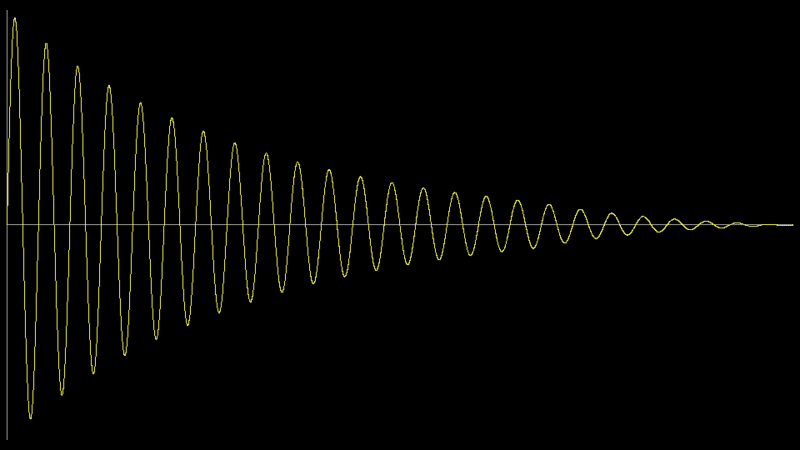Tom.G
Please ignore this post. I missed ln19 so this does not apply. Tom

20 x = c*x - s*y ' complex multiply (c+sj)*(x+yj)
21 y = c*y + s*z ' = (cx-sy),(cy+sx)j

ln 20: "x' is not used
ln21: "z" is undefined

Last edited:
Try this sort of thing...
Code:
' digital raindrop oscillator
Dim As Double x, y ' sound phasor is a complex number = real, imag
Dim As Double t = 0.25 ' length of sound recording in seconds
Dim As Double r = 44100 ' sound sample rate per second
Dim As Double f = 100 ' frequency Hz
Dim As Double e = 0.9997 ' (1-decay) per sample
Const As Double TwoPi = 8 * Atn( 1 )

Dim As Double c, s ' the complex rotator sets frequency and decay rate
c = e * Cos( TwoPi * f / r )
s = e * Sin( TwoPi * f / r )

Dim As Integer n = t * r ' number of samples
Dim As Double a( 0 To n ) ' the sound record

x = 1 ' start of the sound phasor
y = 0
For i As Integer = 0 To n
Dim As Double z = x ' temporary x
x = c*x - s*y ' complex multiply (c+sj)*(x+yj)
y = c*y + s*z ' = (cx-sy),(cy+sx)j
a( i ) = y ' sound output sequence
Next i

' kill the exponential tail
Dim As Integer m = n * 0.33
For i As Integer = n - m To n
a( i ) *= ( n - i ) / m
Next i
View attachment 284816
Hi,
what is Atn( 1 )?

is this VB?

Baluncore
ln 20: "x' is not used
ln21: "z" is undefined
@Tom.G Do you understand how to rotate a vector by using a complex multiply.

x is the real part of the vector = phasor, y is the imaginary part. (x+yj) is a complex number.
x and y are recomputed in each pass of the loop.

I ring the tuned circuit with a step function by setting x = 1; then I take y as the output so it rises from zero without too much click. The risetime is the time the raindrop takes to stop. Then the fabric rings and the sound is absorbed.

z was defined in line 19 as a temporary store of the old x since it is being overwritten in line 20 but is needed again in line 21.
what is Atn( 1 )?

is this VB?
Atn(1) is arctangent(1.00) = 45°=π/4 radians; 8 * atn(1) = 2⋅π

Yes it is also VB, I wrote it as Freebasic, you can call it pseudocode.
It is trivial to rewrite it in any language that takes your fancy.

@Tom.G Do you understand how to rotate a vector by using a complex multiply.

x is the real part of the vector = phasor, y is the imaginary part. (x+yj) is a complex number.
x and y are recomputed in each pass of the loop.

I ring the tuned circuit with a step function by setting x = 1; then I take y as the output so it rises from zero without too much click. The risetime is the time the raindrop takes to stop. Then the fabric rings and the sound is absorbed.

z was defined in line 19 as a temporary store of the old x since it is being overwritten in line 20 but is needed again in line 21.

Atn(1) is arctangent(1.00) = 45°=π/4 radians; 8 * atn(1) = 2⋅π

Yes it is also VB, I wrote it as Freebasic, you can call it pseudocode.
It is trivial to rewrite it in any language that takes your fancy.
Hi thanks for all of this
I have a few questions,

1) Why is your number of sample = sample * Frequency ?
I am asking because I was getting a run time error
that says, 'Index was out of range.'

here:
// kill the exponential tail
int m = (int) (n * 0.33);
for (int i = n - m; i <= n; i++)
{
a1 *= (n - i) / (double) m;
}

2) to fix that error I does this. Does it look right to you?
Rain Fall:
  x = 1; // start of the sound phasor
y = 0;

int m = (int)(n * 0.33);

for (int i = 1; i <n; i++)
{
double z = x; // temporary x
x = c * x - s * y; // complex multiply (c+sj)*(x+yj)
y = c * y + s * z; // = (cx-sy),(cy+sx)j

// kill the exponential tail
if (i >= n - m)
{
y *= (n - i) / (double) m;
}

}

Here is how it come out:

Here is how the graph looks: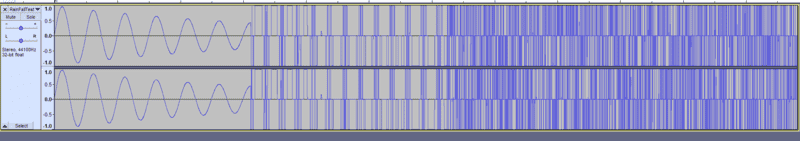something went wrong
lol
any ideas?

#### Attachments

Try this sort of thing...
Code:
' digital raindrop oscillator
Dim As Double x, y ' sound phasor is a complex number = real, imag
Dim As Double t = 0.25 ' length of sound recording in seconds
Dim As Double r = 44100 ' sound sample rate per second
Dim As Double f = 100 ' frequency Hz
Dim As Double e = 0.9997 ' (1-decay) per sample
Const As Double TwoPi = 8 * Atn( 1 )

Dim As Double c, s ' the complex rotator sets frequency and decay rate
c = e * Cos( TwoPi * f / r )
s = e * Sin( TwoPi * f / r )

Dim As Integer n = t * r ' number of samples
Dim As Double a( 0 To n ) ' the sound record

x = 1 ' start of the sound phasor
y = 0
For i As Integer = 0 To n
Dim As Double z = x ' temporary x
x = c*x - s*y ' complex multiply (c+sj)*(x+yj)
y = c*y + s*z ' = (cx-sy),(cy+sx)j
a( i ) = y ' sound output sequence
Next i

' kill the exponential tail
Dim As Integer m = n * 0.33
For i As Integer = n - m To n
a( i ) *= ( n - i ) / m
Next i
View attachment 284816

So I made a few Changes and now my file looks like this :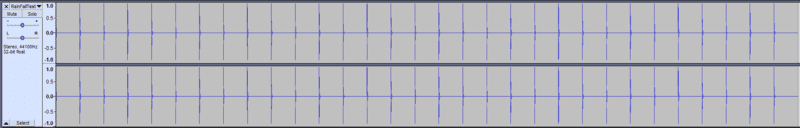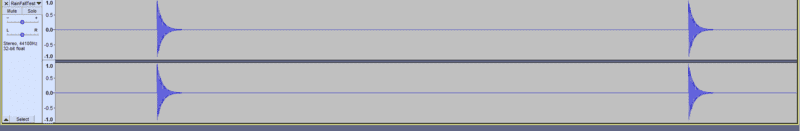it sounds more like a slow drum now:

So I am using Naudio, It is a free audio playing lib (https://markheath.net/post/introducing-naudio-net-audio-toolkit)

anyhow, I am given this function:
int Read(byte[] buffer, int offset, int sampleCount)
{

}

I have to fill in the buffer that is of size sampleCount.

so you are doing :
n = ( t * r);
where r is 44100 sampling rate .
I set my waveformat to 44100 sampleRate and 2 channels.

I am getting a buffer size of 52920 when I play is live
but then I save the file I get a different buffer size of 1411200.

so the save file is sounds different then when I play it live.
The frequency is faster live.

Normally I keep my n as a class variable to I do not break my signal and that works,
but I am not sure how I would do that in this case.
any Ideas ?

Baluncore
Everyone will have a different ideal raindrop sound that will bring back memories of the shelter they were in at one time. Your ear will hear and believe what you expect.
This is experimental. I did not listen to it before I posted the code.
Reduce the recording time to 0.125 sec.
Raise the centre frequency = resonant frequency of the cabin roof.
Change to using x as the output so you get a distinct impact like a hail stone.
Adjust the damping time to suit.
Normally I keep my n as a class variable to I do not break my signal and that works,
but I am not sure how I would do that in this case.
any Ideas ?
I chose a convenient order of computation. You may have to solve for parameters in a different order.

Tom.GI missed ln.19.•